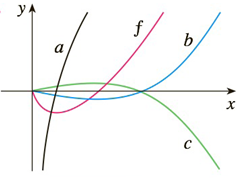Chapter 3.9, Problem 45E

Chapter
Section
Textbook Problem

# 45-46 The graph of a function f is shown. Which graph is an antiderivative of f and why?To determine

To Identify:

Graph of antiderivative of f(x)

Explanation

Explanations:

1) Concept:

Use the concept of increasing and decreasing to decide the graph.

2) Given:

The function f(x) cuts x-axis, and at that point f(x) changes its sign from negative to positive,

3) Calculation:

When the graph f

### Still sussing out bartleby?

Check out a sample textbook solution.

See a sample solution

#### The Solution to Your Study Problems

Bartleby provides explanations to thousands of textbook problems written by our experts, many with advanced degrees!

Get Started

#### Find dy/dx by implicit differentiation. 20. tan(xy)=y1+x2

Single Variable Calculus: Early Transcendentals, Volume I

#### CHECKPOINT 2. Solve the system.

Mathematical Applications for the Management, Life, and Social Sciences

#### True or False: is a convergent series.

Study Guide for Stewart's Single Variable Calculus: Early Transcendentals, 8th